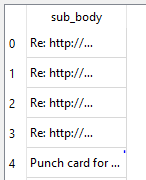# Connect to mongoDB and display data in table

• Hi everyone,
I am creating an application that connects to MongoDB and displays the data in table view/ table widget. I am able to get the data and convert it to a dataframe using a pushbutton. but not able to view the data. here is my code,

``````    self.retranslateUi(MainWindow)
QtCore.QMetaObject.connectSlotsByName(MainWindow)
self.pushButton.clicked.connect(self.button_click)
self.table.clicked.connect(self.returndata)

def retranslateUi(self, MainWindow):
_translate = QtCore.QCoreApplication.translate
MainWindow.setWindowTitle(_translate("MainWindow", "MainWindow"))
self.pushButton.setText(_translate("MainWindow", "PushButton"))

@pyqtSlot( )
def button_click(self):
db = client['mail_test']
test = db.mail_test
test = pd,DataFrame(list(test.find()))
print(test)

@pyqtSlot( )
def returndata(self):
test = self.table.text()``````

• not able to view the data

What does that mean? Where are you showing the data?

• I need to show data in tablewidget, for which the function is table

• @praneshpk
So if you want to use a `QTableWidget` you would need to copy the returned data into the appropriate items.

Usually a `QTableView` is a better choice if you have your own data model. `QTableWidget` has its own internal model which you cannot change.

``````test = pd,DataFrame(list(test.find()))
``````

I am not familiar that Python has this syntax? [I thought the parentheses for tuples were mandatory.] And what is `pd` anyway since it's not declared? [This may not matter now.]

• python is fine. What I am asking is how to display some text in tableview when button is pressed

• @praneshpk
Look at QTableView Class and (particularly) Model/View Programming for your choices and examples.

Only you know how the data/model returned by, say, `DataFrame(list(test.find()))` looks.

• It is working. Thanks.
Now I'm able to get data from MongoDB and display it in table view. But the size is not adjusted.
I have attached the code and screenshot. I have tried resizing. It is not working

``````    self.tableView.setSizeAdjustPolicy(QtWidgets.QAbstractScrollArea.AdjustToContents)
self.tableView.resizeRowsToContents()
self.tableView.resizeColumnsToContents()
``````• @praneshpk
I don't know about `setSizeAdjustPolicy(QtWidgets.QAbstractScrollArea.AdjustToContents)`, that is to do with the tableview as a whole. I don't think I have ever used that. `resizeColumnsToContents()` ought work. But when do you call it? Must be after the table data is shown. I assume your whole table has enough room, and you're just trying to resize a column within it; or does your whole table need expanding to fit that column?

• Whole table has fixed size and contents are not visible, I need to resize so that I can see the contents easily.

• Hi,

Is your table view inside a layout ?

• No, it is inside stacked pages widget

• I'm getting contents in a fixed size, I need this to be changed manually or dynamically.

``````def button_click(self):
db = client['mail_test']
collection = db.mail_test
df = pd.DataFrame(list(collection.find()))
df = df.filter(["sub_body"])
print(df)

model = PandasModel(df)
self.tableView.setModel(model)
``````

and the model file is ,

``````from PyQt5 import QtCore
import pandas as pd

class PandasModel(QtCore.QAbstractTableModel):
def __init__(self, df = pd.DataFrame(), parent=None):
QtCore.QAbstractTableModel.__init__(self, parent=parent)
self._df = df

if role != QtCore.Qt.DisplayRole:
return QtCore.QVariant()

if orientation == QtCore.Qt.Horizontal:
try:
return self._df.columns.tolist()[section]
except (IndexError, ):
return QtCore.QVariant()
elif orientation == QtCore.Qt.Vertical:
try:
# return self.df.index.tolist()
return self._df.index.tolist()[section]
except (IndexError, ):
return QtCore.QVariant()

def data(self, index, role=QtCore.Qt.DisplayRole):
if role != QtCore.Qt.DisplayRole:
return QtCore.QVariant()

if not index.isValid():
return QtCore.QVariant()

return QtCore.QVariant(str(self._df.iloc[index.row(), index.column()]))

def setData(self, index, value, role):
row = self._df.index[index.row()]
col = self._df.columns[index.column()]
if hasattr(value, 'toPyObject'):
# PyQt4 gets a QVariant
value = value.toPyObject()
else:
# PySide gets an unicode
dtype = self._df[col].dtype
if dtype != object:
value = None if value == '' else dtype.type(value)
self._df.set_value(row, col, value)
return True

def rowCount(self, parent=QtCore.QModelIndex()):
return len(self._df.index)

def columnCount(self, parent=QtCore.QModelIndex()):
return len(self._df.columns)

def sort(self, column, order):
colname = self._df.columns.tolist()[column]
All of the stuff you show is for the model. Sizing is dealt with by the view. Whatever you want/need to do about that should be in the `QTableView`.